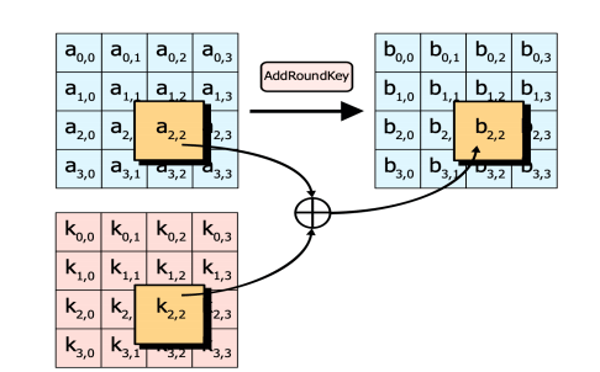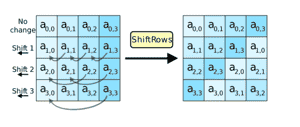Related Tags

network security

# What are Substitution-Permutation networks?

Anusheh Zohair Mustafeez

## Overview

A Substitution-Permutation network or an SP network is a class of block ciphers that consist of rounds of a repeated series of mathematical operations. SP networks form the basis of the infamous AES algorithm.## Operations in an SP network

As the figure above shows, the plaintext is passed to an SP network to produce a ciphertext. This is done through rounds, each of which consists of three main operations:

1. Addition of the round key
2. Substitution of bits
3. Permutation of bits

Let’s study these three steps in more detail.

### Addition of the round key

Each round in an SP network has a round key. Round keys are retrieved from the expansion of one secret key passed to the network at the start. At the start of each round, the text is XORed with the respective round key. This ensures that the ciphertext can only be decrypted by someone who has the round keys.### Substitution of bits

Next, the bits of the text are substituted among themselves. Since SP networks are used in block ciphers, the text is arranged as blocks. The block text bytes are substituted based on rules dictated by predefined S-boxessubstitution boxes.### Permutation of bits

Finally, comes the permutation step. In this step, bits in the block text are mixed around. One example of such mixing is in AES, where all rows except the first are shifted by one. This is shown below:Note: The substitution and permutation boxes are not kept hidden in SP networks. Only the round keys are kept secret for security.

RELATED TAGS

network security

CONTRIBUTOR

Anusheh Zohair Mustafeez